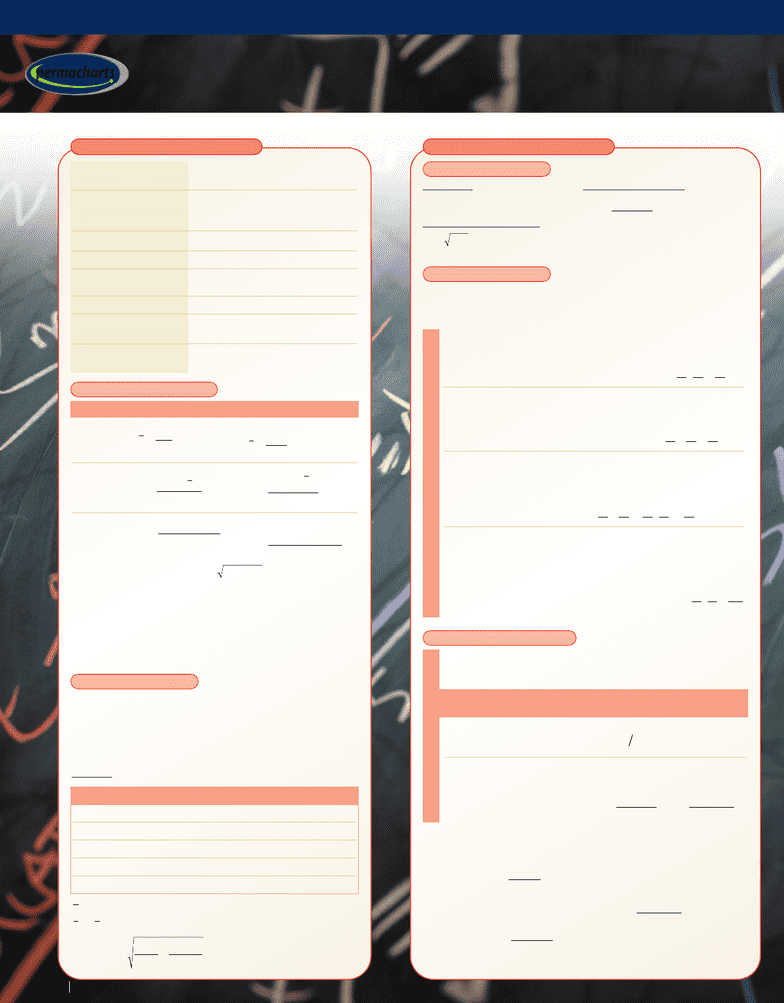# Statistics - Reference Guides

8593 views4 pagesStatistics
Statistics
SAMPLES PROBABILITY THEORY
PROPERTIES OF SAMPLED DATA
Parameter Ungrouped Data Data Grouped in kClasses
Note: Standard Deviation = s=
Range of sample:
xmax xmin
Mode: Most common
value of x
Median: Middle value or
mean of two middle values
Coefficient of variation:
V= (s/x
)100
Quartiles Q25, Q50, Q75:
Values that divide data set into
4 equal parts
Interquartile range: Q75 Q25
• Rationalize xiby first subtracting a convenient number m
(often the median or mode) followed by division by a
suitable number c(often the class size)
• New data set uiyields mean x
and standard deviation sx
of original set:
x
= cu
+ mand sx= csu
Example
60–62 5 61 –2 –10 20
63–65 18 64 –1 –18 18
66–68 42 67 (m) 000
69–71 27 70 1 27 27
72–74 8 73 2 16 32
Notes: u
is the mean of
the new set x
is the
mean of the original set
sxis the standard
deviation of original set
Mean
Variance
Variance
(alternate)
THE CODE METHOD
variance
Class Frequency, fixiui= (xim)/cf
iuifiui2
ufun
xcum
scscfu
n
fu
nn
ii
xu ii ii
== =
=+= +=
=⋅ = =
∑∑
// .
(. ) .
()
().
15 100 0 15
3015 67 6745
11
297
22
FACTORIAL FUNCTIONS
nnn n!()( )()()()=−−12321
n Factorial
nnne
n
!(/)2
π
Stirling’s Approximation
n
r
n
rnr
n
=
=
=
!
!( )! 0
0
01
Binomial Coefficients
Note: 0! = 1
PROBABILITY THEORY
Probability P(S) of “success” = number of events considered
successes (S) total number of possible events (n)
Probability P(F) of “failure” = 1 P(S)
• The probability P(A«B) that two independent events A and B
will both occur, simultaneously or in succession, is:
Example: The probability of drawing a queen
and a heart from two separate decks is:
• The combined probability P(A»B) that one or the other of two
mutually exclusive events Aand Bwill occur is:
Example: The probability of drawing a
queen or a jack from a single deck is:
• The combined probability P(A»B) that one or the other or
both of the two events Aand B will occur is:
Example: The probability of drawing a queen or a heart
or the queen of hearts
from a single deck is:
• The probability that event Boccurs given that event Ahas
already occurred is called the conditional probability P(B|A)
• The probability of the sequence occurring is:
Example: The probability of drawing a queen
and a jack in succession from a single deck is:
Rule 4 Rule 3 Rule 2 Rule 1
PA PB()()
PA PB()()+
PA PB PA B()()( )+−∩
PAB PA PB A()()(|)= ⋅
PA B( )∪= +
()
=
4
52
13
52
4
52
13
52
4
13
PA B( )∪= + =
4
52
4
52
2
13
PA B( )∩= =
4
52
13
52
1
52
PERMUTATIONS & COMBINATIONS
Rule 3 Rule 2 Rule 1
• The number of permutations (= arrange ments including
changes in order) of ndistinct objects
taken all at a time is:
• Number of permutations
of ndistinct objects taken
rat a time is:
• Number of combinations
(= arrangements omitting
changes in order) of ndistinct
objects taken rat a time is:
nr
Pnnr= −!()!
nr
Cn
r
n
rnr
=
=
!
!( )!
nr r
Pn=
nr
Cnr
r
nr
rn
=+−
=+−
1
1
1
()!
!( )!
Without With Repetition
Repetition of Objects
Example: Given the following 3 objects (lJD), n= 3, r= 2
(two taken at a time)
32
32
3
32 6
3
23 2 3
P
C
==
=
==
=
!
()!
!
!( )!
●✤ ✤✪ ●✪
✤● ✪✤ ✪●
●✤ ●✪ ✪✤
32 2
32
39
321
23 1 6
P
C
==
=+−
=
()!
!( )!
to the outcome at left
✤✤ ✪✪ ●●
Without
Repetition
With Repetition of Objects
xx
n
i
=
x
xf
n
ii
k
=
1
sxx
n
i
22
1
=
()
s
fx x
n
ii
k
2
2
1
1
=
()
snx x
nn
ii
222
1
=
()
()
s
nxf xf
nn
ii ii
kk
2
2
1
2
1
1
=
()
nn
Pn=!
Population Totality of objects, individuals, and
events
Sample Part of population chosen for
measurement and observation
• Number of sample values is n
Class Subdivision of sample values xi
Class mark, xiMidpoint value of a class
Class size, cDifference between succes sive
lower or upper class limits
Class frequency, fNumber of sample values in a class
Relative frequency, f/n Class frequency divided by total
number of values n
Cumulative frequency Total number of values less than
given value
STATISTICS • 1-55080-620-31
TM
permacharts
l e a r n r e f e r e n c e r e v i e w
www.permacharts.com
Unlock document

This preview shows page 1 of the document.
Unlock all 4 pages and 3 million more documents.